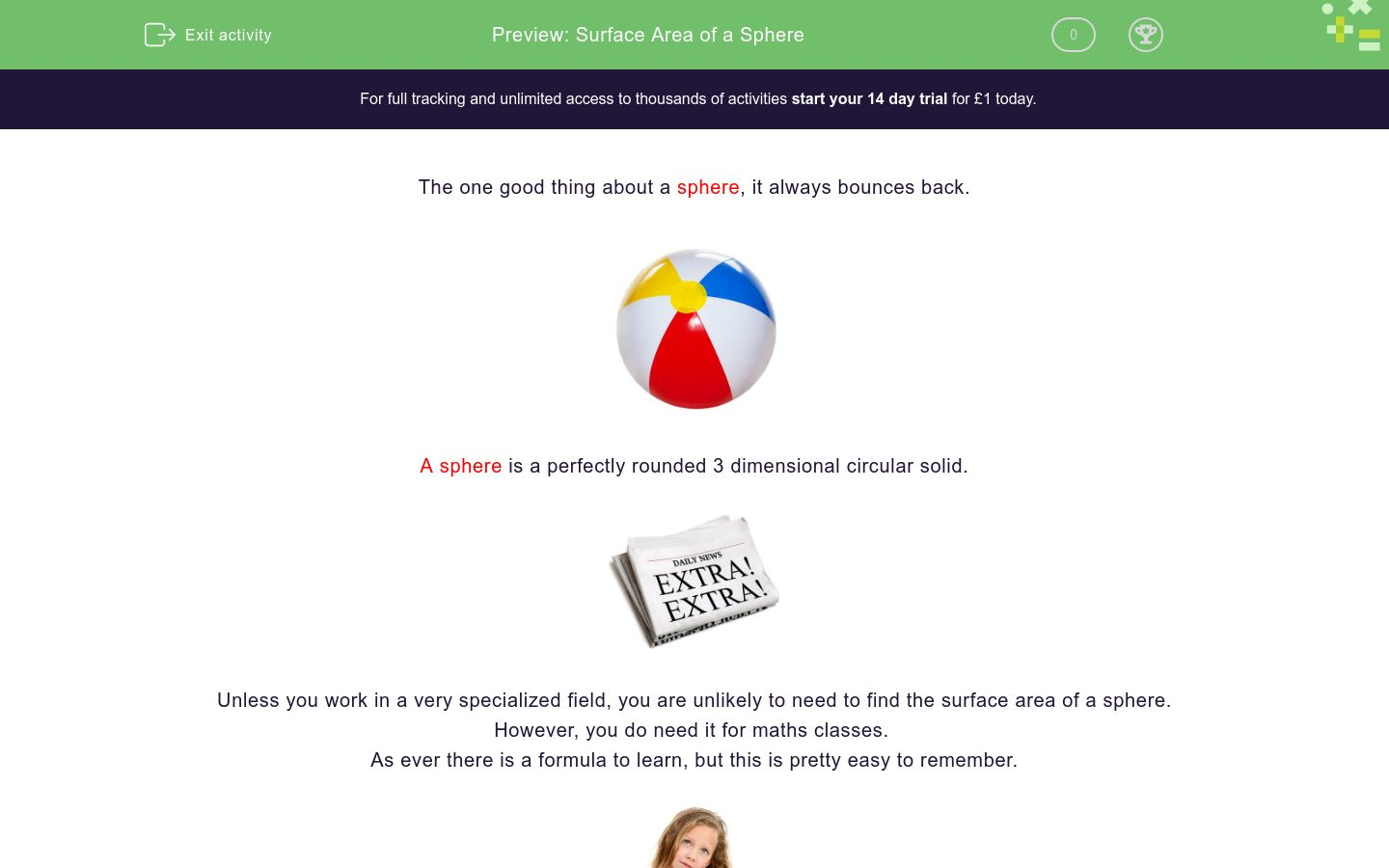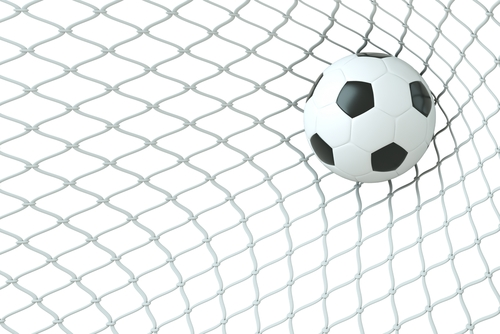# Surface Area of a Sphere

In this worksheet students will learn the formula for finding the surface area of a sphere and be able to apply it.Key stage:  KS 4

GCSE Subjects:   Maths

GCSE Boards:   OCR, AQA, Eduqas, Pearson Edexcel

Curriculum topic:   Mensuration, Geometry and Measures

Curriculum subtopic:   Area Calculations, Mensuration and Calculation

Difficulty level:### QUESTION 1 of 10

The one good thing about a sphere, it always bounces back.A sphere is a perfectly rounded 3 dimensional circular solid.Unless you work in a very specialized field, you are unlikely to need to find the surface area of a sphere.

However, you do need it for maths classes.

As ever there is a formula to learn, but this is pretty easy to remember.Can you remember the formula for the area of a circle?

Surface area of a sphere = 4 x Π x r x r or can be written as 4Πr²

As you can see it is just the formula for finding the area of a circle with the number 4 in front.

Remember r is the radius, which is measured from the centre of the circle to the edge.

Can you remember the value of Π?  3.142 or 3.14 is normally used in calculations.

It is best for this level of work to use a scientific calculator as you can put everything into the calculator at once without trying to work things out first.

Let's get started!Find the surface area of this sphere.

Surface area = 4 x Π x r x r

4 x Π x 8 x 8 = 804 cm²

As we are working with area we always answer in units²

This is all there is to it, you just have to learn the formula.

Let roll on...

What is the formula needed to find the surface area of a sphere?

4π x r cm²

1/3π x r cm²

4/3π x r x r x r cm²

4π r x r cm²

Find the surface area of this sphere with a radius of 4 cm.4π x r cm²

1/3π x r cm²

4/3π x r x r x r cm²

4π r x r cm²4π x r cm²

1/3π x r cm²

4/3π x r x r x r cm²

4π r x r cm²Calculate the surface area of this sphere.  The radius is 3 cm.

113.10 cm²

113.50 cm²

112.90 cm²

121.90 cm²

Find the surface area of this sphere.113.10 cm²

113.50 cm²

112.90 cm²

121.90 cm²This football fits into a cube box with a side of 30 cm.

Calculate the surface area of the football.

113.10 cm²

113.50 cm²

112.90 cm²

121.90 cm²

Can you work out which sphere goes with the correct calculation for surface area?

## Column B

Surface area of a sphere, radius 7 cm
2463.01 cm²
Surface area of a sphere, radius 5 cm
615.75 cm²
Surface area of a sphere, diameter 16 cm
804.35 cm²
Surface area of a sphere, diameter 28 cm
314.16 cm²

Calculate the surface area of this hemisphere.## Column B

Surface area of a sphere, radius 7 cm
2463.01 cm²
Surface area of a sphere, radius 5 cm
615.75 cm²
Surface area of a sphere, diameter 16 cm
804.35 cm²
Surface area of a sphere, diameter 28 cm
314.16 cm²Hemisphere A                                   Hemisphere B

 395.84 cm² 1089.56 cm² 904.78 cm² 1017.88 cm² 508.94 cm² Surface area of hemisphere A Surface area of hemisphere B Difference in surface areaThe surface area of this beach ball is 600 cm²

 395.84 cm² 1089.56 cm² 904.78 cm² 1017.88 cm² 508.94 cm² Surface area of hemisphere A Surface area of hemisphere B Difference in surface area
• Question 1

What is the formula needed to find the surface area of a sphere?

4π r x r cm²
EDDIE SAYS
It is just a case of learning it! Remember as the object is round, think about finding the area of a circle and put the number four in front
• Question 2

Find the surface area of this sphere with a radius of 4 cm.EDDIE SAYS
We know the radius is 4 cm. so4 x Π x r x r = 4 x Π x 4 x 4 = 201.06 rounded to 2 d.p You have to round working with spheres don't you !!!
• Question 3EDDIE SAYS
Practice applying the formula makes perfect. 4 x Π x 9 x 9 = 1017.88 cm² Are you coming around to the idea.!!!
• Question 4Calculate the surface area of this sphere.  The radius is 3 cm.

113.10 cm²
EDDIE SAYS
Are you bouncing with joy because you have learnt the formula. You don't have to groan that loudly... 4 x π x 3 x 3 = 113.10 cm² ( to 2 d.p)
• Question 5

Find the surface area of this sphere.EDDIE SAYS
Okay, I know that was a little sneaky. Just checking your concentration hadn't rolled away. You were given a measurement across the front of the sphere. You needed to halve it to get the radius. 4 x Π x 6 x 6 = 452.39 cm²
• Question 6This football fits into a cube box with a side of 30 cm.

Calculate the surface area of the football.

EDDIE SAYS
What!? I hear you cry. There is no radius given. This is to get you to think outside of the box. You know a cube has sides all the same length. So to get the radius just have the side of the cube. The ball is going to fit in without any room to spare. 4 x Π x 15 x 15 = 2827 cm²
• Question 7

Can you work out which sphere goes with the correct calculation for surface area?

## Column B

Surface area of a sphere, radius ...
615.75 cm²
Surface area of a sphere, radius ...
314.16 cm²
Surface area of a sphere, diamete...
804.35 cm²
Surface area of a sphere, diamete...
2463.01 cm²
EDDIE SAYS
Did your eagle eye spot the two diameters given. Remember to halve them to get the radius. Then just apply the formula.
• Question 8

Calculate the surface area of this hemisphere.EDDIE SAYS
This shouldn't phase you. A hemisphere is half of a sphere. Apply the formula as usual and divide by two at the end. 4 x Π x 26 x 26 = 8494.866 & divide2; = 4247.43 (4247 to the nearest whole number)
• Question 9Hemisphere A                                   Hemisphere B

 395.84 cm² 1089.56 cm² 904.78 cm² 1017.88 cm² 508.94 cm² Surface area of hemisphere A Surface area of hemisphere B Difference in surface area
EDDIE SAYS
Just when you think you have it sussed, there is always the question that wants more. Find the surface area of each hemisphere by applying the formula and then dividing by 2. 4 x Π x r x r ÷ 2 The word difference suggests a subtraction 904.78 - 508.94 = 395.84 cm²
• Question 10The surface area of this beach ball is 600 cm²

EDDIE SAYS
The beloved curved ball. You always have the information you need. You just need to be able to work backwards doing the opposite mathematical operation. 4 x Π x r x r = surface area So, Surface area of 600 ÷ π ÷ 4 then √ the answer 600 ÷ π ÷ 4 = 47.746 .... √ 47.746 = 6.91 (7 to the nearest whole number)
---- OR ----

Sign up for a £1 trial so you can track and measure your child's progress on this activity.

### What is EdPlace?

We're your National Curriculum aligned online education content provider helping each child succeed in English, maths and science from year 1 to GCSE. With an EdPlace account you’ll be able to track and measure progress, helping each child achieve their best. We build confidence and attainment by personalising each child’s learning at a level that suits them.

Get started